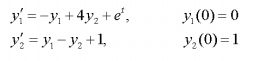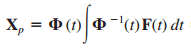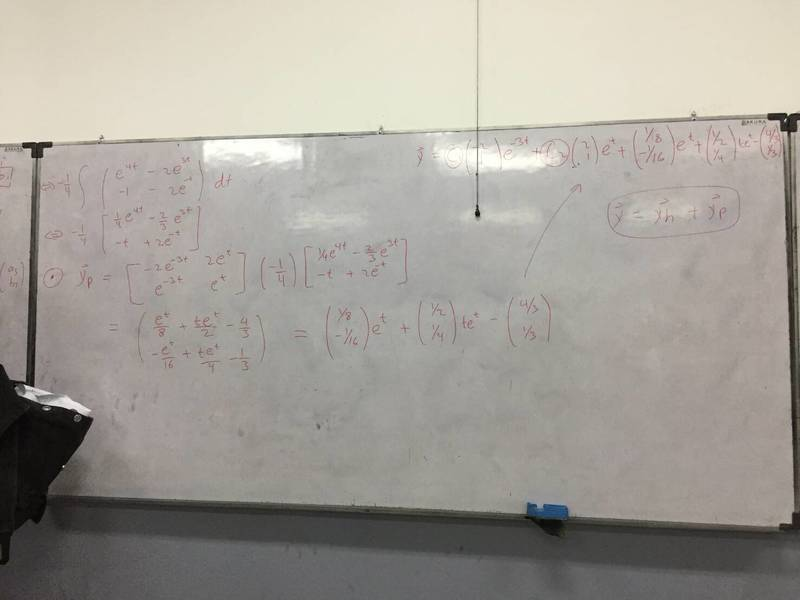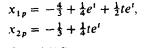# Variation of Parameters

• terryds

## Homework StatementDetermine the y_particular solution

## Homework Equations## The Attempt at a Solution

I've tried this for hours but still don't get the correct value.
This is what I get:The question is the same as the one I found from http://www4.ncsu.edu/eos/users/s/slc/www/COURSES/File2.pdf (Example 6.11.2).
The correct solution is as follows.I get the coefficient for e^t is 1/8 and 1/16, but it says that the correct one is 1/4 and 0.

[UPDATE]

It turns out that the method of parameter variation REALLY yields DIFFERENT PARTICULAR SOLUTION from method of undetermined coefficients,

Undetermined Coefficient: http://www4.ncsu.edu/eos/users/s/slc/www/COURSES/File2.pdf (Example 6.11.2)

Variation of Parameters: http://www.wolframalpha.com/input/?i=solve+x'+=+-x+++4y+++e^t,+y'+=+x-y+1

So which one is wrong, the undetermined coefficient or variation of parameter for this problem??
Or is it possible that those all are correct answers?

Last edited:

I've not looked at your shown work but am responding to your updated comment directly. Recall that your general solution is the particular solution plus the range of all possible homogeneous solutions. Thus adding any of the homogeneous solutions to the particular solution yields another particular solution. They are both right if the particular plus homogeneous solutions yields the same (correct) set of general solutions.

It is a generalization of the process of integration... to find the indefinite integral you find an anti-derivative (particular) and then add the homogeneous solution to d/dx = 0 namely an arbitrary constant. Note that any anti-derivative may be used as they all differ by a constant. For the more general linear diffeq. all of your particular solutions differ by some solution to the homogeneous eqn.

## Homework Statement

View attachment 212489
Determine the y_particular solution

## Homework Equations

View attachment 212490

## The Attempt at a Solution

I've tried this for hours but still don't get the correct value.
This is what I get:
View attachment 212491

The question is the same as the one I found from http://www4.ncsu.edu/eos/users/s/slc/www/COURSES/File2.pdf (Example 6.11.2).
The correct solution is as follows.
View attachment 212492

I get the coefficient for e^t is 1/8 and 1/16, but it says that the correct one is 1/4 and 0.

[UPDATE]

It turns out that the method of parameter variation REALLY yields DIFFERENT PARTICULAR SOLUTION from method of undetermined coefficients,

Undetermined Coefficient: http://www4.ncsu.edu/eos/users/s/slc/www/COURSES/File2.pdf (Example 6.11.2)

Variation of Parameters: http://www.wolframalpha.com/input/?i=solve+x'+=+-x+++4y+++e^t,+y'+=+x-y+1

So which one is wrong, the undetermined coefficient or variation of parameter for this problem??
Or is it possible that those all are correct answers?